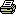# Part 9 - Incoming!

Copy and paste the code below into Form1

1. Private Sub MoveFlies()
2.    Randomize(Now.Ticks)
3.    For Each F As Fly In mobjFlies
4.       Dim NX, NY As Single
5.       Displacement(F.Position.X, F.Position.Y, F.Speed, F.Direction, NX, NY)
6.       If NX > Me.ClientSize.Width - 1 Then F.Direction += CSng(Rnd() * Math.PI)
7.       If NX < 0 Then F.Direction += CSng(Rnd() * Math.PI)
8.       If NY > Me.ClientSize.Height - 1 Then F.Direction += CSng(Rnd() * Math.PI)
9.       If NY < 0 Then F.Direction += CSng(Rnd() * Math.PI)
10.       F.Position.X = CInt(NX)
11.       F.Position.Y = CInt(NY)
12.       Dim FoundScent As Boolean = False
13.       For Each R As Receptor In F.Receptors
14.            Displacement(F.Position.X, F.Position.Y, R.Distance, R.Direction +F.Direction, NX, NY)
15.             For Each S As Scent In mobjScents
16.               If CircleCircle(S.Position.X, S.Position.Y, S.Strength, NX, NY,R.Distance) Then
17.                  F.Direction += R.Direction
18.                  ' Try using this line instead
19.                  'F.Direction += ((R.Direction * 0.9F) + (Rnd() * (R.Direction * 0.2F)))
20.                   FoundScent = True
21.                   Exit For
22.                End If
23.             Next
24.       Next
26.    Next
27. End Sub

Finally after all that setup we can begin to code some AI. The MoveFlies method is at the heart of this application. First it re-seeds the random number generator, and then begins to process each of the flies in the flies collection.

The NX and NY variables are used to store the next location that the fly will be moving to. A call to the Displacement method is made and stores the new fly position in the NX and NY variables. If the new position is outside of the bounds of the form then a new random direction is given to the fly so that it does not try to constantly escape from view.

The FoundScent variable will store weather or not a scent was detected by a receptor. It then proceeds to process each receptor. To determine the location of the receptor on screen a call is made to the Displacement method again. Now that the location of the receptor is known it begins to check each scent in an effort to determine if the receptor collides with it. To determine if a collision takes place a call to the CircleCircle method is made.

The next line of code is how the fly knows what direction to move to, and how it is able to fallow a trail of scent objects.

1. F.Direction += R.Direction

It takes the direction the fly is currently facing and adds the direction that the receptor is facing. Because the direction of the receptors are relative the fly will then be facing in the general direction of the scent.

Now that a scent has been detected we can set the FoundScent variable to true and exit the scent checking loop.

After all receptors have been processed it checks if a scent was found and if so calls the flies update method. The Fly.Update method checks to see if the fly has changed direction within the last half second, and if not changes the flies direction to a new random direction.

Next: Conclusion | 1 | 2 | 3 | 4 | 5 | 6 | 7 | 8 | 9 | 10 | 11

Tutorial by:

### cbx

Date: 2006 Apr 18#### NavigatePrinter Friendly